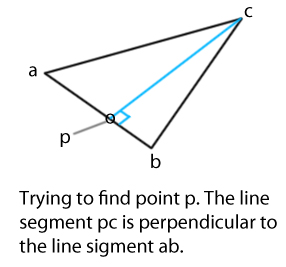# Finding a specific point on the edge of a triangle

Hello everyone! So, we have a triangle composed by points a, b, and c in 3D space. The point we are trying to find is point p and it is on the line segment between a and b. However, the one other defining characteristic of point p is that the line segment it creates with point c is perpendicular to a and b. Please take a look at the attached picture for greater clarity.A solution I can’t seem to see through was to get the plane’s normal (n1) by cross product ca with cb.

n1 = ca cross cb

Then, cross product this normal with ab to get all vectors that are perpendicular to ab (including pc).

n2 = n1 cross ab

Because pc is parallel to n2, it would seem that the vector n2 could be a key to solving this puzzle. Additionally, the dot product of n2 and pc would be the positive or negative magnitude of both vectors multiplied together.

n2 dot pc = (+/-)(magnitude of n2)(magnitude of pc)

Also, when we dot product pc with n1, the result should also be zero (because pc is perpendicular to n1):

n1 dot pc = 0

I’m pretty sure both of the dot products are instrumental in finding out the x, y, and z values of p. Maybe another solution would simply be by setting the line pc equal to the line ab. Or, a combination of setting the lines equal to each-other (getting the intersection point) and these cross/dot product identities. Can someone help me out here and remind me how to do this?

Thanks for your time and help!

This is the answer – which I got from stack exchange after I posted this question there too.

It turns out that point p is the projection of ac onto ab! And, there is a projection function in Unity that solves this problem marvelously. So:

1. add the projection of ac onto ab to point a